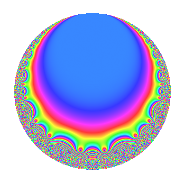Properties

 Label 24.6.aLevel 24 Weight 6 Character orbit a Rep. character $$\chi_{24}(1,\cdot)$$ Character field $$\Q$$ Dimension 3 Newforms 3 Sturm bound 24 Trace bound 5

Related objects

Defining parameters

 Level: $$N$$ = $$24 = 2^{3} \cdot 3$$ Weight: $$k$$ = $$6$$ Character orbit: $$[\chi]$$ = 24.a (trivial) Character field: $$\Q$$ Newforms: $$3$$ Sturm bound: $$24$$ Trace bound: $$5$$ Distinguishing $$T_p$$: $$5$$

Dimensions

The following table gives the dimensions of various subspaces of $$M_{6}(\Gamma_0(24))$$.

Total New Old
Modular forms 24 3 21
Cusp forms 16 3 13
Eisenstein series 8 0 8

The following table gives the dimensions of the cuspidal new subspaces with specified eigenvalues for the Atkin-Lehner operators and the Fricke involution.

$$2$$$$3$$FrickeDim.
$$+$$$$+$$$$+$$$$1$$
$$+$$$$-$$$$-$$$$1$$
$$-$$$$+$$$$-$$$$1$$
Plus space$$+$$$$1$$
Minus space$$-$$$$2$$

Trace form

 $$3q$$ $$\mathstrut -\mathstrut 9q^{3}$$ $$\mathstrut +\mathstrut 98q^{5}$$ $$\mathstrut +\mathstrut 24q^{7}$$ $$\mathstrut +\mathstrut 243q^{9}$$ $$\mathstrut +\mathstrut O(q^{10})$$ $$3q$$ $$\mathstrut -\mathstrut 9q^{3}$$ $$\mathstrut +\mathstrut 98q^{5}$$ $$\mathstrut +\mathstrut 24q^{7}$$ $$\mathstrut +\mathstrut 243q^{9}$$ $$\mathstrut +\mathstrut 20q^{11}$$ $$\mathstrut -\mathstrut 102q^{13}$$ $$\mathstrut -\mathstrut 198q^{15}$$ $$\mathstrut -\mathstrut 266q^{17}$$ $$\mathstrut -\mathstrut 5364q^{19}$$ $$\mathstrut +\mathstrut 1944q^{21}$$ $$\mathstrut +\mathstrut 4904q^{23}$$ $$\mathstrut +\mathstrut 2061q^{25}$$ $$\mathstrut -\mathstrut 729q^{27}$$ $$\mathstrut -\mathstrut 10422q^{29}$$ $$\mathstrut +\mathstrut 1920q^{31}$$ $$\mathstrut +\mathstrut 9252q^{33}$$ $$\mathstrut +\mathstrut 26256q^{35}$$ $$\mathstrut -\mathstrut 1662q^{37}$$ $$\mathstrut -\mathstrut 16398q^{39}$$ $$\mathstrut -\mathstrut 23202q^{41}$$ $$\mathstrut -\mathstrut 13068q^{43}$$ $$\mathstrut +\mathstrut 7938q^{45}$$ $$\mathstrut +\mathstrut 3216q^{47}$$ $$\mathstrut +\mathstrut 42315q^{49}$$ $$\mathstrut -\mathstrut 22050q^{51}$$ $$\mathstrut -\mathstrut 64846q^{53}$$ $$\mathstrut -\mathstrut 11592q^{55}$$ $$\mathstrut +\mathstrut 7164q^{57}$$ $$\mathstrut +\mathstrut 51236q^{59}$$ $$\mathstrut +\mathstrut 37098q^{61}$$ $$\mathstrut +\mathstrut 1944q^{63}$$ $$\mathstrut +\mathstrut 38396q^{65}$$ $$\mathstrut -\mathstrut 11364q^{67}$$ $$\mathstrut +\mathstrut 1800q^{69}$$ $$\mathstrut -\mathstrut 32264q^{71}$$ $$\mathstrut +\mathstrut 24510q^{73}$$ $$\mathstrut -\mathstrut 48807q^{75}$$ $$\mathstrut +\mathstrut 37920q^{77}$$ $$\mathstrut +\mathstrut 39120q^{79}$$ $$\mathstrut +\mathstrut 19683q^{81}$$ $$\mathstrut -\mathstrut 57524q^{83}$$ $$\mathstrut -\mathstrut 199068q^{85}$$ $$\mathstrut +\mathstrut 165186q^{87}$$ $$\mathstrut +\mathstrut 32046q^{89}$$ $$\mathstrut -\mathstrut 9264q^{91}$$ $$\mathstrut -\mathstrut 71136q^{93}$$ $$\mathstrut -\mathstrut 130040q^{95}$$ $$\mathstrut -\mathstrut 180954q^{97}$$ $$\mathstrut +\mathstrut 1620q^{99}$$ $$\mathstrut +\mathstrut O(q^{100})$$

Decomposition of $$S_{6}^{\mathrm{new}}(\Gamma_0(24))$$ into irreducible Hecke orbits

Label Dim. $$A$$ Field CM Traces A-L signs $q$-expansion
$$a_2$$ $$a_3$$ $$a_5$$ $$a_7$$ 2 3
24.6.a.a $$1$$ $$3.849$$ $$\Q$$ None $$0$$ $$-9$$ $$-34$$ $$-240$$ $$+$$ $$+$$ $$q-9q^{3}-34q^{5}-240q^{7}+3^{4}q^{9}+\cdots$$
24.6.a.b $$1$$ $$3.849$$ $$\Q$$ None $$0$$ $$-9$$ $$94$$ $$144$$ $$-$$ $$+$$ $$q-9q^{3}+94q^{5}+12^{2}q^{7}+3^{4}q^{9}+\cdots$$
24.6.a.c $$1$$ $$3.849$$ $$\Q$$ None $$0$$ $$9$$ $$38$$ $$120$$ $$+$$ $$-$$ $$q+9q^{3}+38q^{5}+120q^{7}+3^{4}q^{9}+\cdots$$

Decomposition of $$S_{6}^{\mathrm{old}}(\Gamma_0(24))$$ into lower level spaces

$$S_{6}^{\mathrm{old}}(\Gamma_0(24)) \cong$$ $$S_{6}^{\mathrm{new}}(\Gamma_0(3))$$$$^{\oplus 4}$$$$\oplus$$$$S_{6}^{\mathrm{new}}(\Gamma_0(4))$$$$^{\oplus 4}$$$$\oplus$$$$S_{6}^{\mathrm{new}}(\Gamma_0(6))$$$$^{\oplus 3}$$$$\oplus$$$$S_{6}^{\mathrm{new}}(\Gamma_0(8))$$$$^{\oplus 2}$$